﻿ Coordinates in 3D Space | JavaScript Chart Documentation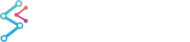SciChart.js JavaScript 3D Charts API > SciChart3D Basics > Coordinates in 3D Space
Coordinates in 3D Space

## Coordinate Systems

### The Left Handed Coordinate System (LHS)

The SciChartSurface3D by default renders a 3D world using the Left Handed Coordinate system or LHS (as is common to WebGL). In the LHS X and Z form the horizontal plane, and Y is always up YDirection=(0,1,0). It is helpful to think of the 3D world as a 2D Chart in X-Y and Z goes ‘into the screen’.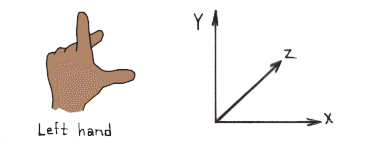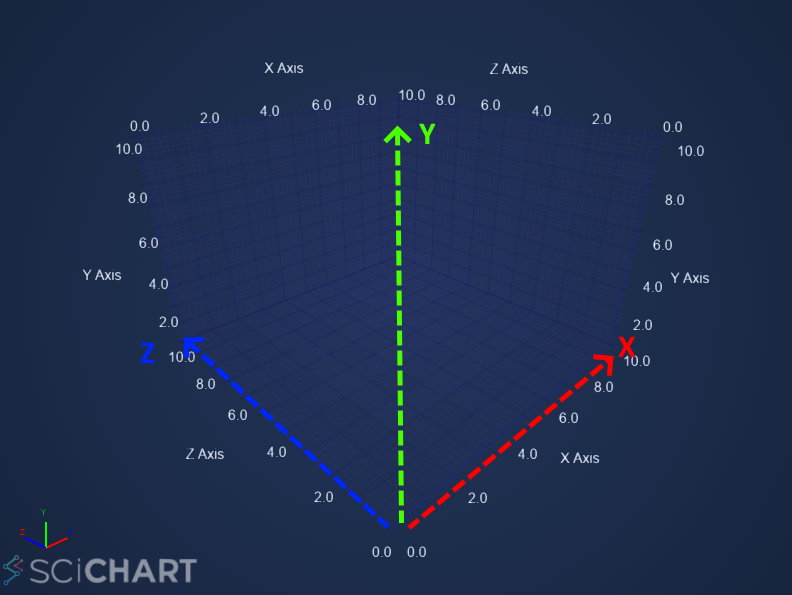Left handed coordinate system. X-Z is a horizontal plane, Y is up.

## World Coordinates

World Coordinates is the term used to describe coordinates in the 3D World. These are the raw X,Y,Z coordinates of a vertex. By default the origin (0,0,0) is in the centre, bottom of the chart.

### WorldDimensions and the Axis Cube

The box in the chart is called the Axis Cube. The AxisCube size is defined by the SciChartSurface3D.WorldDimensions property.

The WorldDimensions is a single Vector3 with X,Y,Z size, but defines the size of a cube as follows: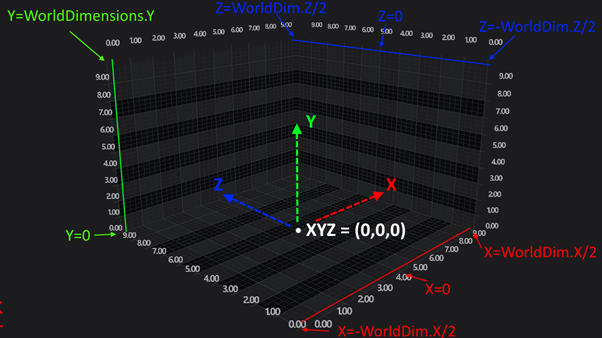How WorldDimensions Relates to the AxisCube in SciChart3D

Therefore…

• The size of the AxisCube in the X Direction extends from -WorldDimensions.X/2 to +WorldDimensions.X/2
• The size of the AxisCube in the Y Direction extends from 0 to +WorldDimensions.Y
• The size of the AxisCube in the Z Direction extends from -WorldDimensions.Z/2 to +WorldDimensions.Z/2
NOTE: By default, SciChart3DSurface.WorldDimensions property is set to X=300, Y=200, Z=300.

### Setting the WorldDimensions Property

To set the WorldDimensions Property, simply use the following code to define a Vector3 (3-component vector):

Setting WorldDimensions
Copy Code
```import { SciChart3DSurface, Vector3 } from "scichart";

// World dimensions can be set at creation of the chart
const { wasmContext, sciChart3DSurface } = await SciChart3DSurface.create(divElementId, {
// Optional dimensions of the axis cube (X,Y,Z) in World coordinates
worldDimensions: new Vector3(300, 200, 300),
});

// Or, it can be set later
sciChart3DSurface.worldDimensions = new Vector3(300, 200, 300);
```

## Data Coordinates

By contrast to WorldCoordinates, which are absolute coordinates in the 3D World, in SciChart 3D there is the concept of Data Coordinates.

All Data in SciChart3D is provided in Data Coordinates. They are converted to World Coordinates by SciChart 3D for display on the chart.

Data Coordinates are measured on an Axis, for example, the YAxis (which is UP) might have a size of 200 in the World Coordinates, but might display a VisibleRange of 0...10. Therefore, Data which falls int he range 0...10 will be spaced on this axis from 0...200 World Coordinates.

The difference between World Coordinates, Data Coordinates is shown in the following diagram: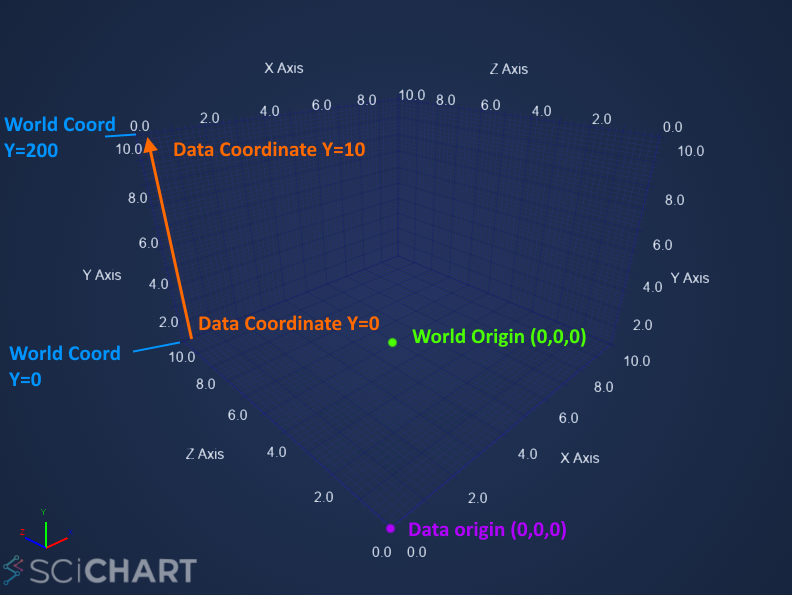How WorldDimensions Relates to the AxisCube in SciChart3D

## Converting from World to Data Coordinates

The conversion between Data Coordinates and World Coordinates is done by the Axis. For example. the following code converts from Data to World Coordinates on the SciChartSurface3D.xAxis.

```// Get the coordinate calculator
const coordCalc = sciChart3DSurface.xAxis.getCurrentCoordinateCalculator();

// Get a world coordinate from data values
const worldX0 = coordCalc.getCoordinate(0);
const worldX10 = coordCalc.getCoordinate(10);

// Convert back to dataValue
const dataValue0 = coordCalc.getDataValue(worldX0);
const dataValue10 = coordCalc.getDataValue(worldX10);

console.log(`Data value at X=\${dataValue0} corresponds to world coordinate X=\${worldX0}`);
console.log(`Data value at X=\${dataValue10} corresponds to world coordinate X=\${worldX10}`);
```